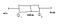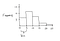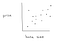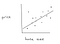# 3 Key Methods for Visualizing Statistical Data

## Understanding the basics of the box plot, histogram, and scatterplotPhoto by Isaac Smith on Unsplash

In our world, few things are as powerful as data. As Engineer W. Edwards Deming put it,

“Without data, you are just another person with an opinion.”

But sometimes, data on its own is not enough to solve the questions we seek to answer. After all, we can not do too much with just a spreadsheet full of numbers. That is why it is extremely important to be able to turn data into something we are able to use. More often than not, this involves transforming said data into a more understandable format. In this article, we will discuss the three of the most common ways to visualize data: the box plot, histogram, and scatterplot.

# The Box Plot

A box plot summarizes and displays five different data points. In increasing numerical order, these are the *minimum value, first quartile, median, third quartile, and *maximum.

These could also be replaced by what are called lower and upper fences*

The box plot takes the shape of a rectangle with a line drawn downwards within the widths of the rectangle, accompanied by two lines coming out of the left and right sides, sometimes called whiskers. Refer to the diagram below with all of the components labeled.screenshot from author

Now, how do we calculate all of these values?

• Minimum: the minimum value of the dataset.
• First Quartile (Q1): the median of the lower half of the dataset.
• Median: the middle number of the dataset; use standard median formula.
• Third Quartile (Q3): the median of the upper half of the dataset
• Maximum: the maximum of the dataset.

When finding the median of either the lower or upper half of the dataset, you may notice a slight problem. Let’s say we have the set of numbers {3,6,7,8,9}. It would not be incorrect to say that the lower half of this dataset is {3,6,7} OR {3,6}. Of course, the median of these two sets of numbers would be different. Luckily, mathematicians have decided that no one method is better than the other. Therefore, we are free to choose how we define “lower half,” as long as we keep it consistent when calculating the third quartile.

In addition, there is also a caveat when dealing with the constructing the ends of the box plot, where the minimum and maximum values are located. We also must consider what are called lower and upper fences. The formula for both of these fences includes the IQR, which is calculated by taking the difference between the third and first quartiles (Q3 - Q1); they are listed below.

• Lower Fence: Q1 - 1.5*IQR
• Upper Fence: Q3 + 1.5*IQR

We end the lower whisker at whichever is higher in numerical value between the lower fence and the minimum. Additionally, we end the upper whisker at whichever is lower in numerical value between the upper fence and the maximum. However, if we cap the whiskers at the lower or upper fence, we still plot the minimum or maximum point.

The box plot is important because we are able to determine outliers and even check for normality. Any value that is outside the bound of the lower or upper fence is defined as an outlier. Additionally, If the distribution of the box plot seems to be relatively symmetrical, we can infer that the distribution of the dataset is approximately normal.

# The Histogram

A histogram helps with visually displaying information using bins. A bin is simply a specific, predetermined range, in which numbers from a dataset will fall under (these bins do not necessarily need to have the same range). Each bin will have a height, which determines the number of values that fall in each bin. The higher the height, the more frequent values from the dataset are present, and the lower the height, the less frequent values from the dataset are present. Take a look at a sample histogram below.screenshot from author

As you can see, this histogram visualizes the data quite well. We can say that the most frequent range of values is between 5–10, and the least frequent range of values is between 20–25 just by looking at the heights of the bars. Furthermore, we can also claim that the dataset is skewed to the right by looking at the general shape of the distribution. Given this, we are able to make claims such as the fact that the mean of the dataset will be greater than the median. In fact, the purpose of creating a histogram is to determine whether the distribution of a dataset is approximately normal, skewed left, skewed right, uniform etc… This step is extremely important in dictating the use of hypothesis tests.

# Scatterplots

A scatterplot plots points of two related variables “in an x-y plane.” In the experimental setting, we use scatterplots when there is one variable that is being controlled that affects another variable. The independent variable, the one that is being altered, is plotted on the horizontal x-axis, and the dependent variable, the one that changes as a result of the independent variable, is plotted along the vertical y-axis.example scatterplot relating house size and price

The main usage of a scatterplot is to attempt to identify types of correlation. Most commonly, we are looking for positive or negative linear relationships, or lack thereof, although there are some instances where we would want to look for quadratic or even cubic relationships. To do this, we implement a processes called linear regression, quadratic regression etc… Linear regression models minimize the sum of the squared errors between the actual and predicted point values, creating a line called the line of best fit.example with possible line of best fit

If we take a look at this sample line of best fit for the link between house size and house price, we can see that it clearly helps us visualize the relationship between the two variables: there is a visibly strong, positive relationship between the house size and price, meaning that we should expect an increase in house size to yield an increase in house price.

# Wrapping Up

The power of visualization is often underestimated. While analysis of raw numbers can get us far, we sometimes miss key details that can only be seen creating a box plot or a histogram, much like how we may only notice to group like terms if they are next to each other in an algebraic expression. Therefore, we should all make the effort to become equally comfortable working with graphs and charts as we are with mathemical equations. Remember, give the numbers a chance to express themselves.

## Geek Culture

Proud to geek out. Follow to join our +500K monthly readers.

## Geek Culture

A new tech publication by Start it up (https://medium.com/swlh).

Written by

## Albert Ming

17 || High School Junior || stats & math## Geek Culture

A new tech publication by Start it up (https://medium.com/swlh).

## More From Medium

Medium is an open platform where 170 million readers come to find insightful and dynamic thinking. Here, expert and undiscovered voices alike dive into the heart of any topic and bring new ideas to the surface. Learn more

Follow the writers, publications, and topics that matter to you, and you’ll see them on your homepage and in your inbox. Explore

If you have a story to tell, knowledge to share, or a perspective to offer — welcome home. It’s easy and free to post your thinking on any topic. Write on Medium